# Comparative Statement | Accounts Class 12

## Comparative Statement

Comparative statement is prepared in order to compare the figures of financial statements for two or more years. It gives an idea about the financial health of the organisation. Every company which wants to estimate its future progress must refer to its past performance. When financial statements of one firm for two or more years are compared then it is known as inter-period comparison. Similarly, when financial statements of two or more firms are compared over a number of years then it is known as inter-firm comparison.

#### Comparative Statement : Example 1:

Following is the information available from the records of AEE Ltd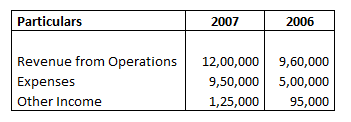Absolute Change and Percentage Change in Revenue from Operations is:

Explanation : –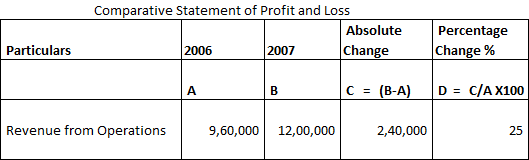Absolute Change = Current year balance – Previous year balance
Absolute Change = 1200000 – 960000 = 240000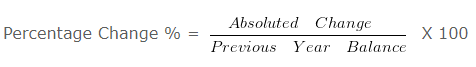Percentage Change % = 240000/960000 X 100

= 25 %

#### Comparative Statement : Example 2

Following is the information available from the records of N Ltd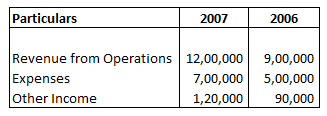Absolute Change and Percentage Change in Expenses is:

Explanation : –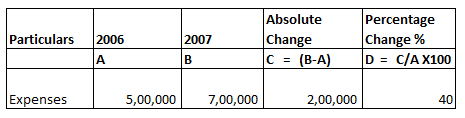Absolute Change = Current year balance – Previous year balance
Absolute Change = 700000 – 500000 = 200000Percentage Change % = 200000/500000 X 100

= 40 %

#### Comparative Statement : Example 3

Following is the information available from the records of ABC Ltd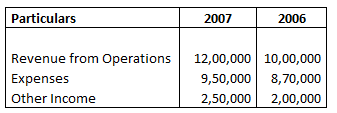Absolute Change and Percentage Change in Other income is:

Explanation : –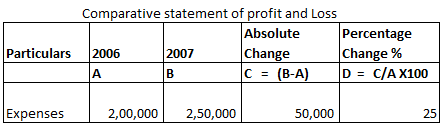Absolute Change = Current year balance – Previous year balance
Absolute Change = 250000 – 200000 = 50000Percentage Change % = 50000/200000 X 100

= 25 %

#### Comparative Statement : Example 4

Following is the information available from the records of One Ltd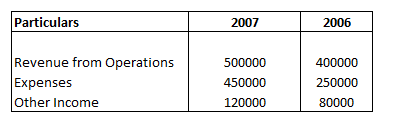Profit before tax is :

Explanation : –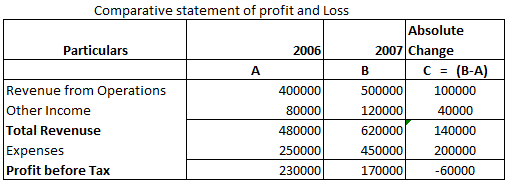Absolute change = Current year balance – Previous year balance
Revenue from Operations = 500000 – 400000 = 100000
Other Income = 120000 – 80000 = 40000
Expenses = 450000 – 250000 = 200000Revenue from Operations = 100000/400000 X 100 = 25

Other Income = 40000/80000 X 100 = 50

Expenses = 200000/250000 X 100 = 80

#### Comparative Statement : Example 5

Following is the information available from the records of ANY LTD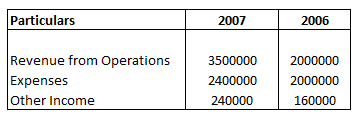Rate of Tax is 20 %
Profit after Tax is :

Explanation : –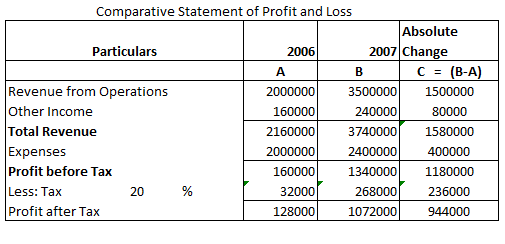Absolute Change = Current year balance – Previous year balance
Revenue from Operations = 3500000 – 2000000 = 1500000
Other Income = 240000 – 160000 = 80000
Expenses = 2400000 – 2000000 = 400000Revenue from Operations = 1500000/2000000 X 100 = 75

Other Income = 80000/160000 X 100 = 50

Expenses = 400000/2000000 X 100 = 20

#### Comparative Statement : Example 6

Following is the information provided by A LTD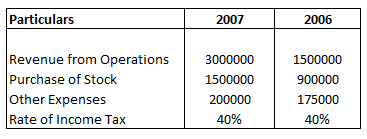Purchase of Stock Percentage to Revenue from Operations is:

Explanation : –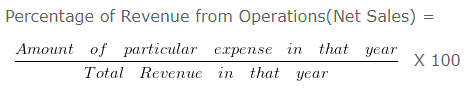Purchase of Stock

2006 = 900000/1500000 X 100

= 60

2007 = 1500000/3000000 X 100

= 50

#### Comparative Statement : Example 7

Following is the information provided by A LTD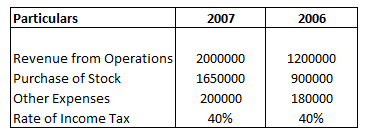Other Expenses Percentage to Revenue from Operations is:

Explanation : –Other Expenses
2006 = 180000/1200000 X 100

= 15

2007 = 200000/2000000 X 100

= 10

#### Comparative Statement : Example 8

Following is the information provided by A LTD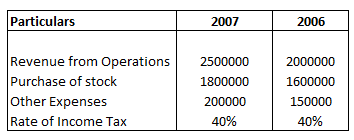Profit after Tax for year 2006 is

Explanation : –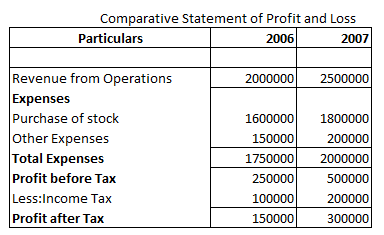#### Comparative Statement : Example 9

Following is the information provided by A LTD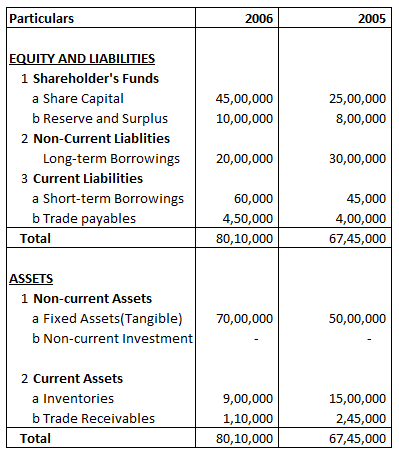Absolute Change and Percentage Change in share capital is

Explanation : –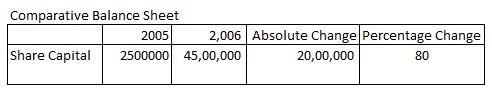Absolute Change = Current year’s figure – Previous year’s figure
= 4500000 – 2500000
= 2000000= 2000000/2500000 X 100

= 80 %

#### Comparative Statement : Example 10

Following is the Balance sheet of AA Ltd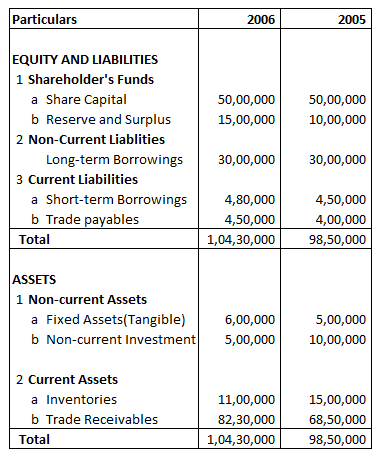Absolute Change and Percentage Change in Reserve and Surplus is:

Explanation : –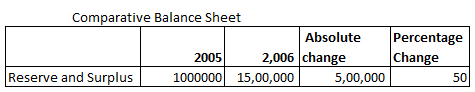Absolute change = Current year’s figure – Previous year’s figure
= 1500000 – 1000000
= 500000= 500000/1000000 X 100

= 50

#### Comparative Statement : Example 11

Following is the Balance sheet of B Ltd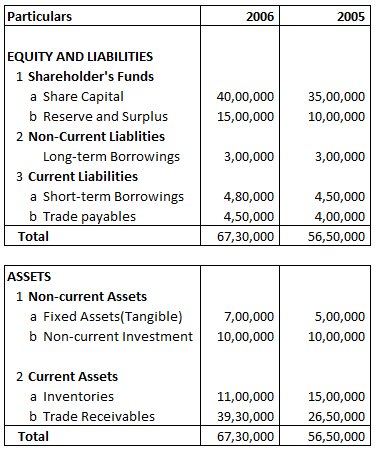Absolute Change and Percentage Change in Long-term Borrowings is:

Explanation : –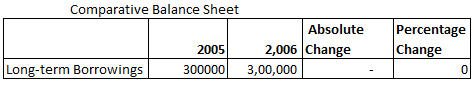Absolute Change = Current year’s figure – Previous year’s figure
= 300000 – 300000
= 0= 0/300000 X 100

= 0

#### Comparative Statement : Example 12

Following is the Balance sheet of A Ltd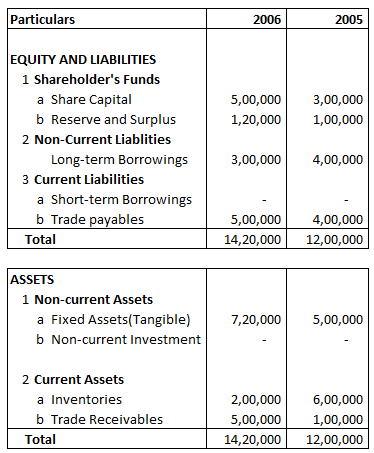Absolute Change and Percentage Change in Trade payable is:

Explanation : –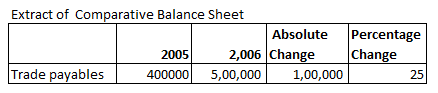Absolute Change = Current year’s figure – Previous year’s figure
= 500000 – 400000
= 100000= 100000/400000 X 100

= 25

#### Comparative Statement : Example 13

Following is the Balance sheet of A Ltd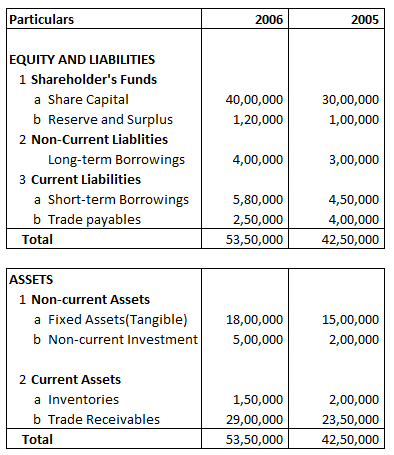Absolute Change and Percentage Change in Fixed Assets(Tangible) is:

Explanation : –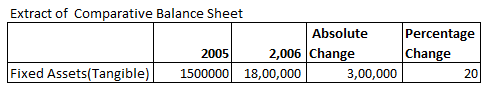Absolute Change = Current year’s figure – Previous year’s figure
= 1800000 – 1500000
= 300000= 300000/1500000 X 100

= 20

#### Comparative Statement : Example 14

Following is the Balance Sheet of AX Ltd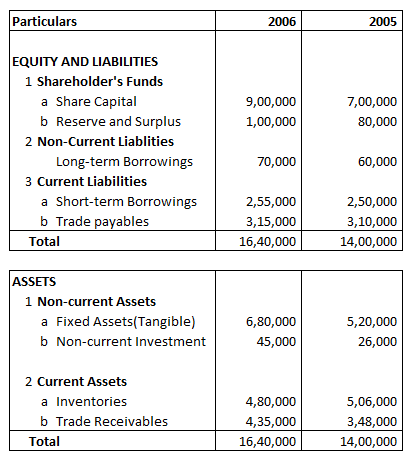Share Capital Percentage of Balance Sheet total for 2005 will be:

Explanation : –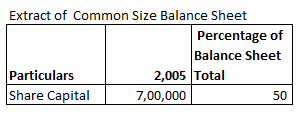Share Capital Percentage of Balance Sheet Total 2005 =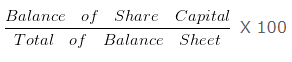= 700000/1400000 X 100

= 50

#### Comparative Statement : Example 15

Following is the Balance Sheet of A Ltd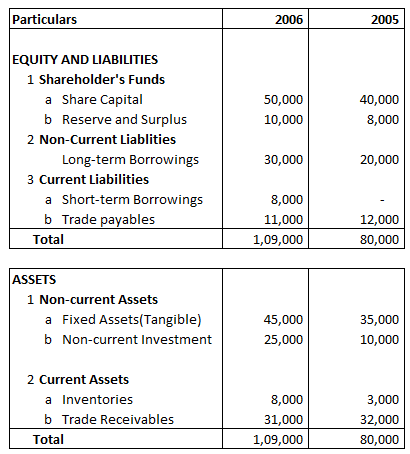Reserve and Surplus Percentage of Balance Sheet total for 2005 will be:

Explanation : –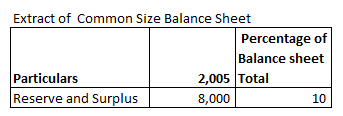Reserve and Surplus Percentage of Balance Sheet Total 2005 =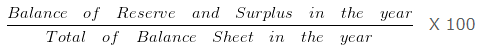= 8000/80000 X 100

= 10

#### Comparative Statement : Example 16

Following is the balance sheet of A Ltd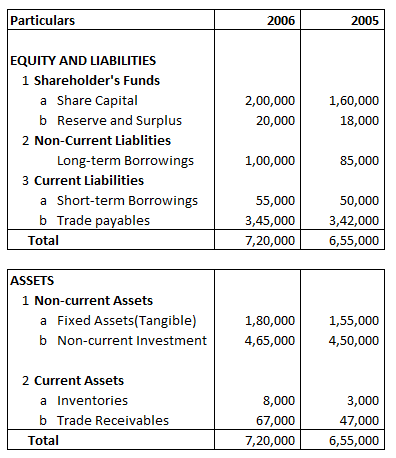Fixed Assets(Tangible) Percentage of Balance Sheet Total for 2006 will be:

Explanation : –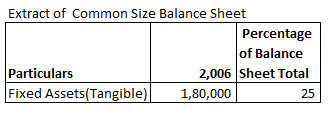Fixed Assets(Tangible) Percentage of Balance Sheet Total 2006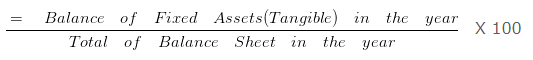= 180000/720000 X 100

= 25

#### Comparative Statement : Example 17

Following is the Balance sheet of AB Ltd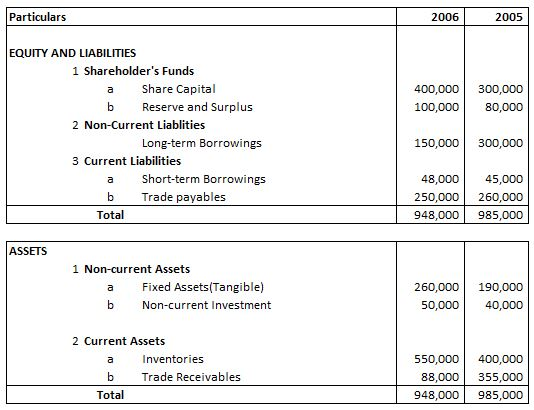Absolute Change and Percentage Change in Inventories is:

Explanation : –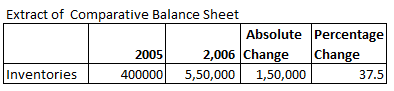Absolute Change = Current year’s figure – Previous year’s figure
= 550000 – 400000
= 150000= 150000/400000 X 100

= 37.5

### 1 thought on “Comparative Statement | Accounts Class 12”

1.Very good explanation sir or mam
I am fan of u if all teachers explain like this than every student will top in accounts
And the way u tell the questions is amazing
I am fan of u

Reply